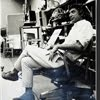## Eugene Ray SDSU 1970'sEugene Ray 1970's. photo credit: Tom Davis

## Thursday, March 21, 2013

### Fwd: EUGENE RAY ARCHITECT'S GIFT> for the sdsu emeritus exhibition ^^^^^^^^^^^^^^^^^^^^^ UNISPHERE as a MISE-EN-SCENE CREATED as a HOLOGRAPHIC SET ^^^^^^^^^^^^^^^^^^^^^^the original 1970 prototype had> >>OMNI-MAX PROJECTION<<> 180 degree film projected on skin >a hologram would improve idea ^^^^^^^^^^^^^^^^^^^^^^THUS EVERYONE COULD LIVE IN> DAVE'S LATEST 3D "SITUATION" paying him a monthly rental fee> the bed located in the >CENTER< WHERE ALL THE BIOTRONICS ARE

+"THE UNIVERSAL SPHEROID"+
(eugene ray architect)<
+2013+
becomes a holographic set based<
on dave fobes latest painting<
^^^^^^^^^^^^^^^^
+THE BED+
located in the very center<
(SEED)<
where energy is the<
+MAXIMUM+
^^^^^^
(>in honor of the sdsu faculty<
exhibition emeritus section<)
^^^^^^^^^^^^^^

+CONCEPT BASED+
on my unisphere concept of<
+1970+
^^^^^^^^^^
+EUGENE RAY ARCHITECT+
+UNISPHERE+
^^^^^^
(esp. jan. 13, 2013 posting<
original unisphere photo)<
^^^^^^^^^^^^

+PLUS+
the current cover article in<
+"FOCUS" MAGAZINE+
march 2013 issue<
^^^^^^^^^
company" in london has a vital<
+HOLOGRAM+
concept that "reality" may be<
+ACTUALLY SIMULATED+
(scientists are intensly working<
to PROVE this fantastc idea<)
^^^^^^^^^^^^^^
+AFTER ALL+
what does an ant comprehend<
of our human/world reality<
^^^^^^^^^^^^^^^

+TONIGHT'S PHOTOS+
(+DAVE'S PAINTING+)
reality in universal spheroid<
^^^^^^^^^^^^^^

synergetic stucture as created<
in my studio at sdsu in the<
using a technique i developed<
manipulating a video camera<
^^^^^^^^^^^^^^^^

#2)              +UNISPHERE+
the double teflon skin openable<
spherical residence w/ dave<
projecting the hologram<
^^^^^^^^^^^^^^
+AFTER+
(>sending my evening blog<
back to me of course<)
^^^^^^^^^^
+JIM BOURKE+
is building the new aquarius<
desert operational center<
^^^^^^^^^^^^^^
+KEVIN & JASMIN+
in the desert nerve center<
+\$\$\$\$\$\$\$\$\$\$\$\$+
overseeing operations<
(brinks trucks galore)<
^^^^^^^^^^
+MOVE OVER MR GATES+
+MR FOBES IS AT THE+
hologram<
+C O N T R O L S+
^^^^^^^

#3)           +R E A L I T Y+
is what god and his associate<
+DAVE FOBES+
are cooking up for you<
^^^^^^^^^^^^
+RALPH BOWMAN+
is in charge of the hollywood<
studio connection and<
+TOM DAVIS+
is in charge of our patents<
^^^^^^^^^^^^^^

#4)         +PLANET EARTH+
with sdsu ed alumni in charge<
+KOTARO-CHIKAKO+
+YOSHIKI+
are in charge of asia and<
+PHILIP+
is in charge of africa while<
+JIMMY & ARATHY+
are in charge of india
^^^^^^^^^^

the french version, shall be my<
+OBSERVATION+
post to enjoy everything from<
^^^^^^^^^^^
+MARIANNE-MARIAN+
^^^^^^^^^^^^
+TOM HART+
+RALPH ROESLING+
sharing profound philosophies<
+SHERRY & NANCIE+
in charge of our decor and<
+FRANK WOLDEN+
and<
+ROGER UTT<
providing the new city<
^^^^^^^^^^
for my first student john messina<
and his wife tania who were w/<
me in new orleans in the<
very beginning of<
^^^^^^^^^